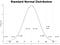# Hello World! 🌎

Hello internet, I am a new user 🐣! Just trying out the medium platform, let's see how it goes! And to include some tips for me for the future 👍.A Lazy Morning — Photo by Steven Lio

# Display some of my favorite Math:

Einstein’s famous Mass-energy equivalence formula 🌌:

From wiki: The formula defines the energy E of a particle in its rest frame as the product of mass m with the speed of light squared ().

The 🔔 Curve :

With the probability density function:

Where μ is the mean or expectation of the distribution and σ is the standard deviation. i.e. variance of σ². It is also known as the Gaussian Distribution or more commonly known as the Normal Distribution.

An example graph of the function:Produced in R (Code below)

To begin a code block in the medium editor:

• Windows/Linux: Ctrl + Alt + 6
• Mac: Command + Option + 6
• or type ``` (triple backtick) on a new line
`#Clear console and environmentrm(list = ls())cat(“\014”)#attach required librarylibrary(ggplot2)library(dplyr)#set mu and sigma parameters for the Normal Curvemu<-0sigma<-1#generate datax<-seq(-4*sigma,4*sigma,0.0001)-0.5y<-dnorm(x,mu,sigma) #density functiondata<-cbind(x=x,y=y) %>% data.frame()xlab<-c(expression(-3*sigma) ,expression(-2*sigma) ,expression(-sigma) ,expression(mu) ,expression(sigma) ,expression(2*sigma) ,expression(3*sigma))p_lab<-pnorm(seq(-3*sigma,3*sigma,sigma),mu,sigma)#Plot:ggplot(data,aes(x,y))+#Plot area settings: theme_classic()+ theme(plot.title=element_text(size=40,face=”bold”,hjust=0.5) ,panel.grid.major.x=element_line(size = (0.2)) ,axis.text.x=element_blank())+ ggtitle(“Standard Normal Distribution”)+ xlab(“x”)+#text for the sigma labels annotate(“text”, x = seq(-3*sigma,3*sigma,sigma), y = rep(-0.005,7), label = xlab, family = “”, fontface = 3, size=8) +#text for the density values at each n*sigma area annotate(“text”, x = c(seq(-3*sigma,3*sigma,sigma)-0.5*sigma,0.5*sigma+3*sigma), y = round(c(p_lab,diff(p_lab),p_lab),1)²+0.05, label = paste0(round(c(p_lab,diff(p_lab),p_lab)*100,1),”%”), family = “”, fontface = 8, size=8) +#display parameter values annotate(“text”, x = rep(2.2*sigma,2), y = c(0.16,0.14), label = c(expression(paste(mu,”=”)), expression(paste(sigma,”=”))), family = “”, fontface = 3, size=8) +  annotate(“text”, x = rep(2.4*sigma,2), y = c(0.164,0.143), label = c(mu,sigma), family = “”, fontface = 3, size=7) + scale_x_continuous(breaks=seq(-3*sigma,3*sigma,sigma),limits=c(-3.5*sigma,3.5*sigma))+ ylab(“Probability Density”)+ scale_y_continuous(labels=scales::percent_format(accuracy=1))+ geom_line()`

Maybe some Linear Algebra:

To find a Plane of best-fit.

Given a data vector with n samples and p parameters:

Where the dependent variable y and the p-size vector of regressors x are assumed to be a linear relationship. Where the error variable ε was modeled such that it is the minimum and ideally it experiences an unobserved random variable. i.e. “noise”.

Then ideally we want to find β where the model has the form:

Or simply

Where

and

Least-squares estimation (a.k.a. Line of best fit):

Since y and x are assumed to be a linear relationship and we would like to find the “best” β which solve the system of equations and to minimize ε. Hence let:

L is called the Loss function, essentially the error term is modeled with the input X, y, β. Since X, y is the original data we want to “fit”, therefore we can find the “best” β at which L(X, y, β) is minimized.

Hence the first derivative of L(X, y, β):

Since X, y is fixed and known and we only interested in the “best” β therefore:

This is the case for the Simple Linear Regression.

This concludes the Hello World! 🌎

Thank you for reading!